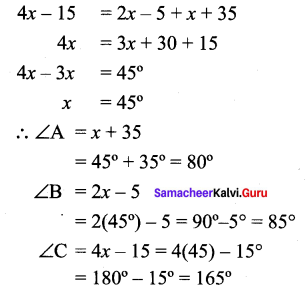# Samacheer Kalvi 9th Maths Solutions Chapter 4 Geometry Ex 4.1

## Tamilnadu Samacheer Kalvi 9th Maths Solutions Chapter 4 Geometry Ex 4.1

Question 1.
In the figure, AB is parallel to CD, find x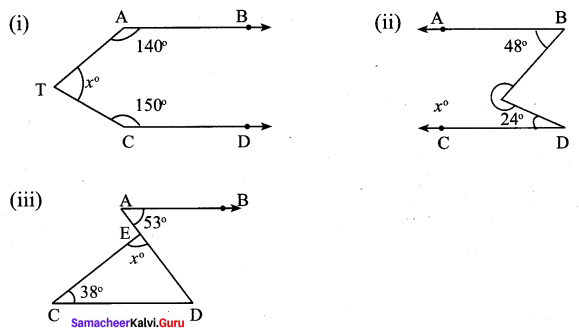Solution:
(i) From the figure
∠1 = 140° (∴ corresponding angles are equal)
∠2 = 40° (∴ ∠1 + ∠2= 180°)
∠3 = 30° (∵ ∠3 + 150= 180°)
∠4 = 110° (∵ ∠2 + ∠3 + ∠4 = 180°)
∴ ∠x = 70° (∵ ∠4 + ∠x = 180°)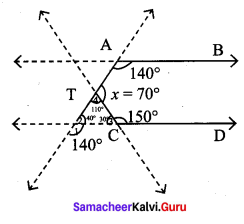(ii) From the figure
∠1 = 48°
∠3 = 108° (∠1 +24° + ∠3 = 180°)
∠4 = 108° (If two lines are intersect, then the vertically the opposite angles are equal)
∠5 = 72° (∵ ∠3 + ∠5 = 180°)
∴ ∠3 + ∠4 + ∠5 = 108° + 108° + 72°
x = 288°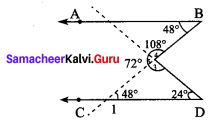(iii) From the figure
∠D = 53° ( ∵ ∠B and ∠D are alternate interior angles)
Sum of the three angles of a triangle is 180°
∠x° = 180°- (38°+ 53°)
= 180°- 91° = 89°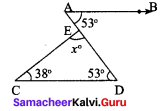Question 2.
The angles of a triangle are in the ratio 1 : 2 : 3, find the measure of each angle of the triangle.
Solution:
Let the angles be x, 2x and 3x respectively.
Sum of the three angles of a triangle = 180°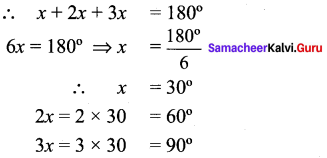The 3 angles of the triangle are 30°, 60°, 90°.

Question 3.
Consider the given pairs of triangles and say whether each pair is that of congruent triangles. If the triangles are congruent, say ‘how’; if they are not congruent say ‘why’ and also say if a small modification would make them congruent: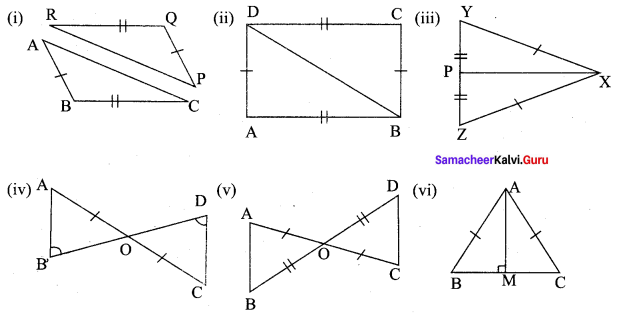Solution:
(i) Consider ∆PQR and ∆ABC
Given, RQ = BC
PQ = AB
∆ABC is not congruent to ∆PQR
If PR = AC, then ∆ABC ≅ ∆PQR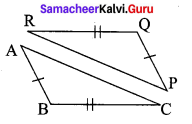(ii) Consider ∆ABD and ∆BCD for the triangles to be congruent.
Given, AB = DC
AD = BC and AB is common side.
∴ By SSS rule ∆ABD ≅ ∆BCD.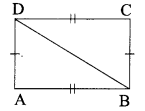(iii) Consider ∆PXY and ∆PXz,
Given, XY = XZ
PY = PZ and PX is common
∴ By SSS rule ∆PXY ≅ ∆PXZ.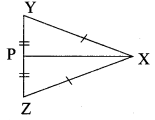(iv) Consider ∆OAB and ∆ODC,
Given, OA = OC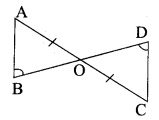∠ABO = ∠ODC and ∠AOB = ∠DOC (vertically opposite angles)
∴ By AAS rule, AOAB = AODC.

(v) Consider ∆AOB and ∆DOC,
Given, AO = OC
OB = OD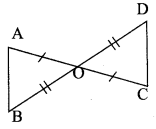and ∠AOB = ∠DOC [vertically opposite angles]
∴ By SAS rule, ∆AOB = ∆DOC.

(vi) Consider ∆AMB and ∆AMC,
Given, AB = AC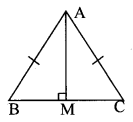∠AMB = ∠AMC = 90°
∴ AM is common.
∴ By RHS rule
∆AMB ≅ ∆AMC.

Question 4.
∆ABC and ∆DEF are two triangles in which AB = DF, ∠ACB = 70°, ∠ABC = 60°; ∠DEF = 70° and ∠EDF = 60°. Prove that the triangles are congruent.
Solution: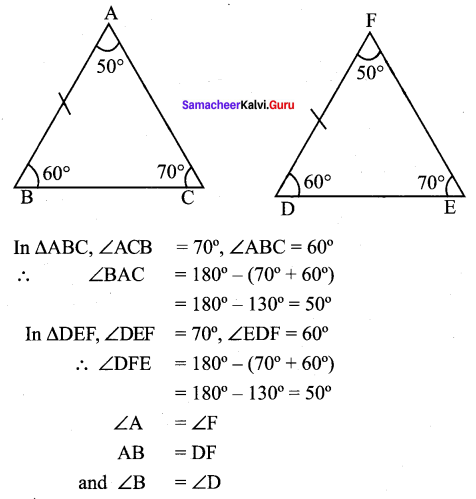∴ By ASA rule ∆ABC ≅ ∆FDE

Question 5.
Find all the three angles of the ∆ABC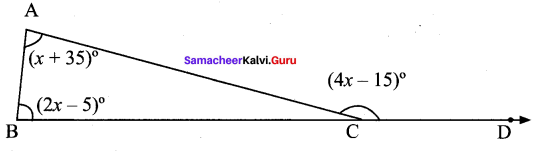Solution:
Exterior angle = Sum of the two opposite interior angles.